#### You may also like### Purr-fection

What is the smallest perfect square that ends with the four digits 9009?### Exhaustion

Find the positive integer solutions of the equation (1+1/a)(1+1/b)(1+1/c) = 2### Instant Insanity

Given the nets of 4 cubes with the faces coloured in 4 colours, build a tower so that on each vertical wall no colour is repeated, that is all 4 colours appear.

# Twin Equivalent Sudoku

##### Age 16 to 18 Challenge Level:

By Henry Kwok
 Twin A Twin B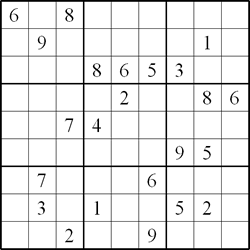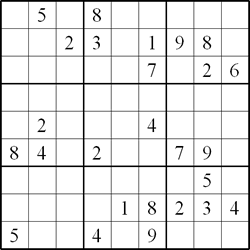Here is a Word document with the two Sudoko grids that you can download for printing.

#### Rules of Twin Equivalent Sudoku

This Sudoku consists of a pair of linked standard Sudoku puzzles each with some starting digits. As usual, the object of this Sudoku is to fill in the whole of each 9x9 grid with digits 1 through 9 so that each row, each column and each block contain all the digits 1 through 9.

Twin B is related to twin A in the following ways:
1. Given that twin A is the original puzzle, we can create an equivalent puzzle from twin A by swapping a horizontal/vertical chute or band of 3 blocks with another horizontal/vertical chute or band of 3 blocks.

For example, an equivalent puzzle (fig 2) is created from fig 1 by swapping the left vertical chute of 3 blocks with the middle vertical chute of 3 blocks.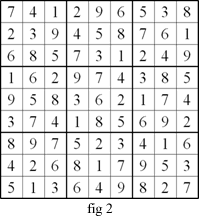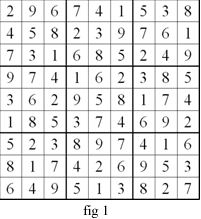Similarly an equivalent puzzle (fig 3) can be created from fig 1 by swapping the top horizontal chute of 3 blocks with the middle horizontal chute of 3 blocks.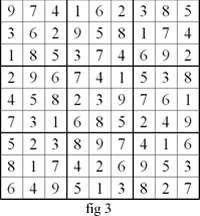2. After swapping the chutes, we can create a third equivalent puzzle (twin B) by renaming all the elements of second equivalent puzzle.

For example, we create an equivalent puzzle (fig 4) by renaming the elements of fig 3 in the following way:

fig 3 $\rightarrow$ fig 4

$1 \rightarrow7$

$2 \rightarrow 6$

$3\rightarrow 1$

$4 \rightarrow 9$

$5 \rightarrow 3$

$6 \rightarrow 2$

$7 \rightarrow 8$

$8 \rightarrow 5$

$9 \rightarrow 4$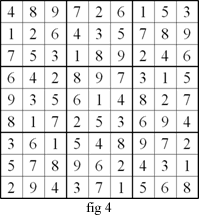The four puzzles (fig 1 to fig 4) are equivalent to one another. Equivalent puzzles can also be created from fig 1 and fig 2 by renaming the elements in the respective grids. Instead of calling fig 1 and fig 4 by the clumsy name of 'twin element-renaming chute-swapping sudoku', we can simply call them 'twin equivalent sudoku'.